Mathematics
Easy

Question

# The product of two rational numbers is 6. If one of the number is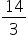, find the other number.

##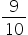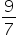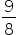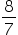Hint:

## The correct answer is:### Product of two rational numbers = 6One rational number =Let the other number be = xso the product of x andis equal to 6The required number is#### With Turito Foundation.#### Get an Expert Advice From Turito.